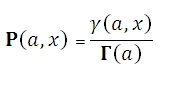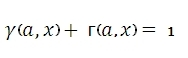# SQL Server lower cdf of the GAMMA distribution function

Updated: 9 August 2010

Use GAMMAP to calculate the regularized gamma function P(a, x). The equation for this function is:WhereSyntax
SELECT [wctStatistics].[wct].[GAMMAP] (
<@A, float,>
,<@X, float,>)
Arguments
@A
a value to be passed to the function. @A is an expression of type float or of a type that can be implicitly converted to float
@X
a value to be passed to the function. @X is an expression of type float or of a type that can be implicitly converted to float
Return Types
float
Remarks
·         If @A <0 or @X is < 0, GAMMAP returns an error.
·         GAMMAP(@A, @X) + GAMMAQ(@A, @X) = 1
Examples

Select
wct.GAMMAP(5,2)

This produces the following result
----------------------
0.0526530172876507

(1 row(s) affected)
--------------GAMMAP 2013-03-05Copyright 2008-2020 Westclintech LLC         Privacy Policy        Terms of Service